# Plotting polytopes in 3D

With gMOIP you can make 3D plots of the polytope/feasible region/solution space of a linear programming (LP), integer linear programming (ILP) model, or mixed integer linear programming (MILP) model. This vignette gives examples on how to make plots given a model with three variables.

library(gMOIP)

We present four examples.

## Example 1

We define the model $$\max \{cx | Ax \leq b\}$$ (could also be minimized) with three variables:

A <- matrix( c(
3, 2, 5,
2, 1, 1,
1, 1, 3,
5, 2, 4
), nc = 3, byrow = TRUE)
b <- c(55, 26, 30, 57)
obj <- c(20, 10, 15)

We load the preferred view angle for the RGL window:

view <- matrix( c(-0.412063330411911, -0.228006735444069, 0.882166087627411, 0, 0.910147845745087,
-0.0574885793030262, 0.410274744033813, 0, -0.042830865830183, 0.97196090221405,
0.231208890676498, 0, 0, 0, 0, 1), nc = 4)

The LP polytope:

loadView(v = view, close = F, zoom = 0.75)
plotPolytope(A, b, plotOptimum = TRUE, obj = obj)

Note in the html file you can zoom/turn/twist the figure with your mouse (rglwidget).

The ILP model with LP and ILP faces:

loadView(v = view)
mfrow3d(nr = 1, nc = 2, sharedMouse = TRUE)
plotPolytope(A, b, faces = c("c","c","c"), type = c("i","i","i"), plotOptimum = TRUE, obj = obj,
argsTitle3d = list(main = "With LP faces"), argsPlot3d = list(box = F, axes = T) )
plotPolytope(A, b, faces = c("i","i","i"), type = c("i","i","i"), plotFeasible = FALSE, obj = obj,
argsTitle3d = list(main = "ILP faces") )

Let us have a look at some MILP models (we use static images). MILP model with variable 1 and 3 integer:

loadView(v = view, close = T, zoom = 0.75)
plotPolytope(A, b, faces = c("c","c","c"), type = c("i","c","i"), plotOptimum = TRUE, obj = obj)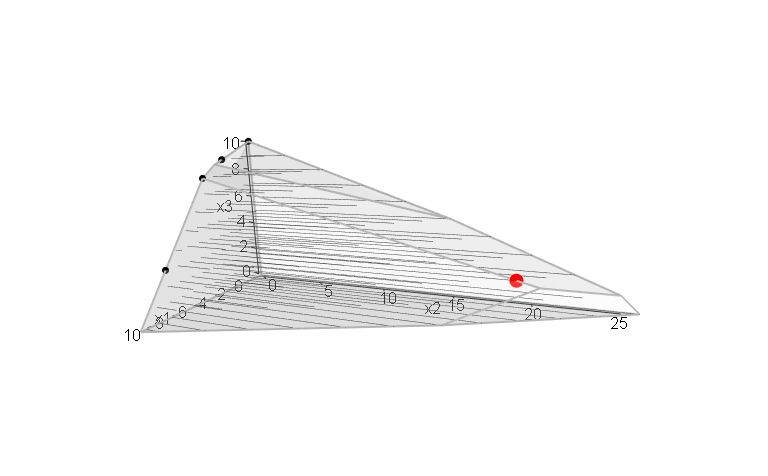MILP model with variable 2 and 3 integer:

loadView(v = view, zoom = 0.75)
plotPolytope(A, b, faces = c("c","c","c"), type = c("c","i","i"), plotOptimum = TRUE, obj = obj)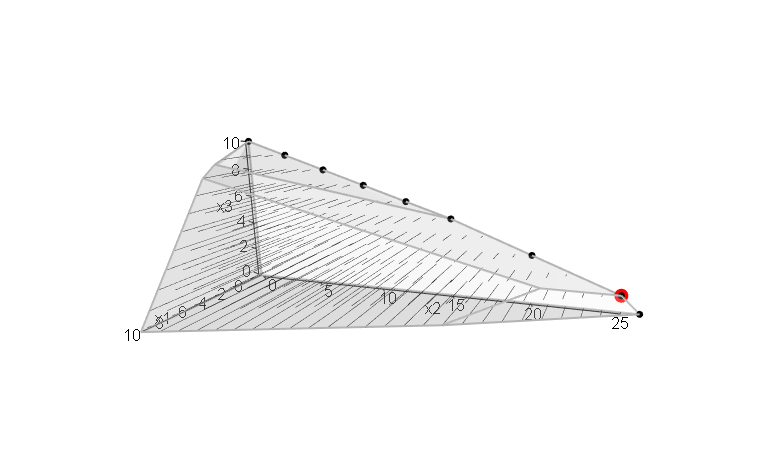MILP model with variable 1 and 2 integer:

loadView(v = view, zoom = 0.75)
plotPolytope(A, b, faces = c("c","c","c"), type = c("i","i","c"), plotOptimum = TRUE, obj = obj)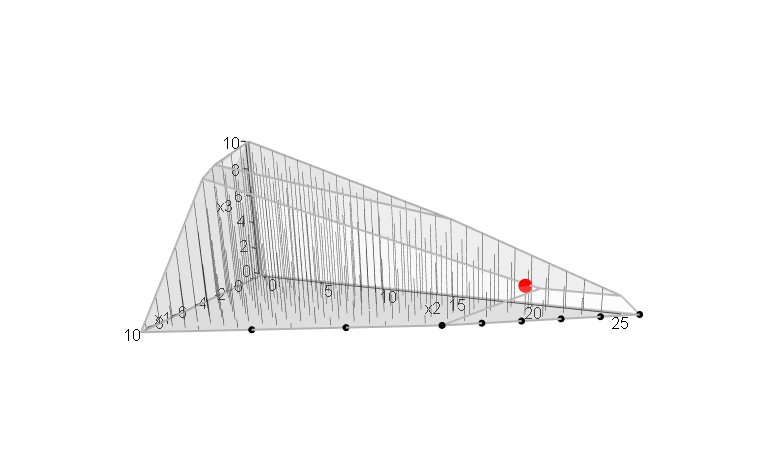MILP model with variable 1 integer:

loadView(v = view, zoom = 0.75)
plotPolytope(A, b, type = c("i","c","c"), plotOptimum = TRUE, obj = obj, plotFaces = FALSE)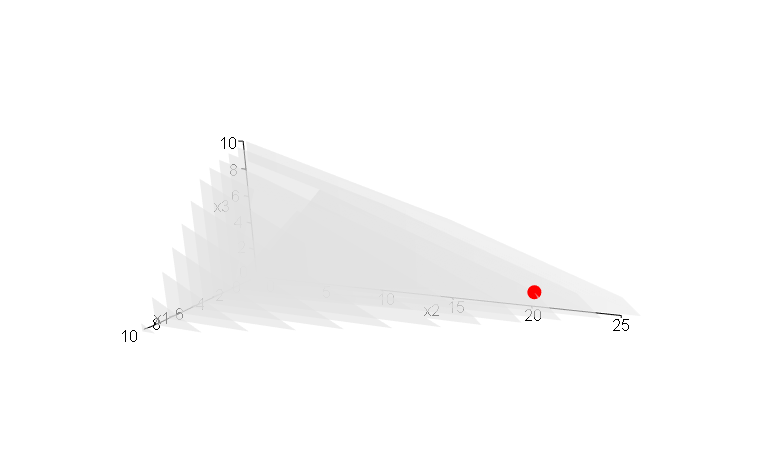MILP model with variable 2 integer:

loadView(v = view, zoom = 0.75)
plotPolytope(A, b, type = c("c","i","c"), plotOptimum = TRUE, obj = obj, plotFaces = FALSE)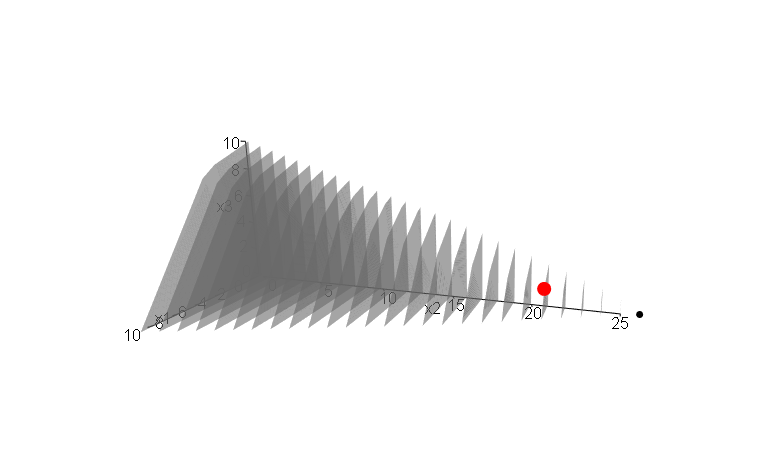MILP model with variable 3 integer:

loadView(v = view, zoom = 0.75)
plotPolytope(A, b, type = c("c","c","i"), plotOptimum = TRUE, obj = obj, plotFaces = FALSE)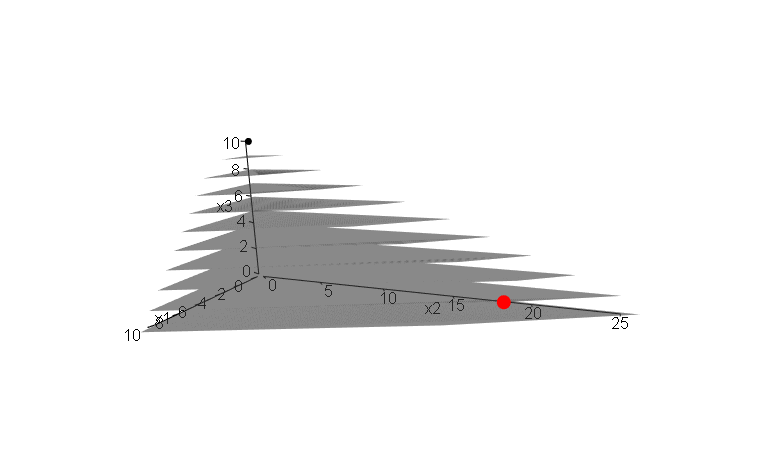## Example 2

We define the model:

A <- matrix( c(
1, 1, 1,
3, 0, 1
), nc = 3, byrow = TRUE)
b <- c(10, 24)
obj <- c(20, 10, 15)

We load the preferred view angle for the RGL window:

view <- matrix( c(-0.812462985515594, -0.029454167932272, 0.582268416881561, 0, 0.579295456409454,
-0.153386667370796, 0.800555109977722, 0, 0.0657325685024261, 0.987727105617523,
0.14168381690979, 0, 0, 0, 0, 1), nc = 4)

The LP polytope:

loadView(v = view, close = F, zoom = 0.75)
plotPolytope(A, b, plotOptimum = TRUE, obj = obj, labels = "coord")

Note in the html file you can zoom/turn/twist the figure with your mouse (rglwidget).

The ILP model with LP and ILP faces:

loadView(v = view)
mfrow3d(nr = 1, nc = 2, sharedMouse = TRUE)
plotPolytope(A, b, faces = c("c","c","c"), type = c("i","i","i"), plotOptimum = TRUE, obj = obj,
argsTitle3d = list(main = "With LP faces"), argsPlot3d = list(box = F, axes = T) )
plotPolytope(A, b, faces = c("i","i","i"), type = c("i","i","i"), plotFeasible = FALSE, obj = obj,
argsTitle3d = list(main = "ILP faces") )

Let us have a look at some MILP models (we use static images). MILP model with variable 1 and 3 integer:

loadView(v = view, close = T, zoom = 0.75)
plotPolytope(A, b, faces = c("c","c","c"), type = c("i","c","i"), plotOptimum = TRUE, obj = obj)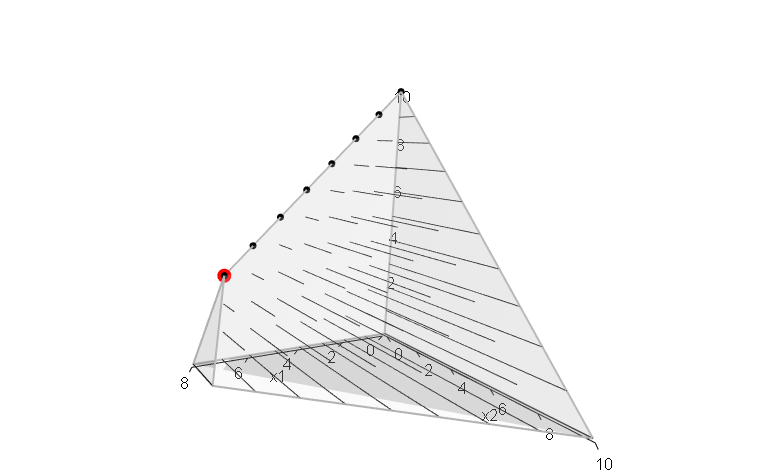MILP model with variable 2 and 3 integer:

loadView(v = view, zoom = 0.75)
plotPolytope(A, b, faces = c("c","c","c"), type = c("c","i","i"), plotOptimum = TRUE, obj = obj)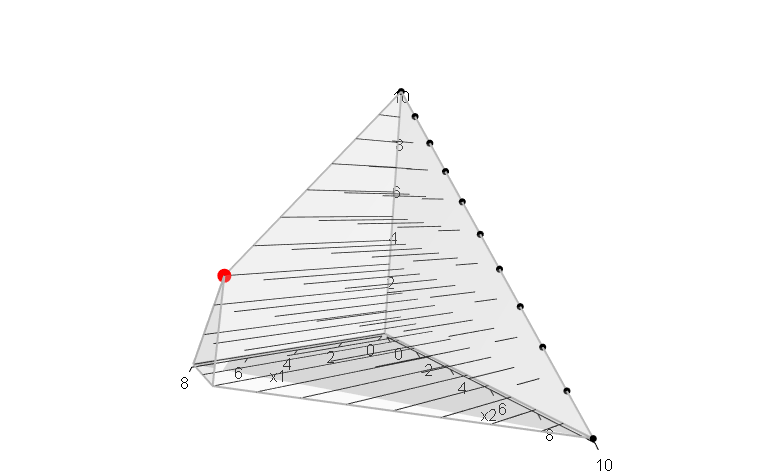MILP model with variable 1 and 2 integer:

loadView(v = view, zoom = 0.75)
plotPolytope(A, b, faces = c("c","c","c"), type = c("i","i","c"), plotOptimum = TRUE, obj = obj)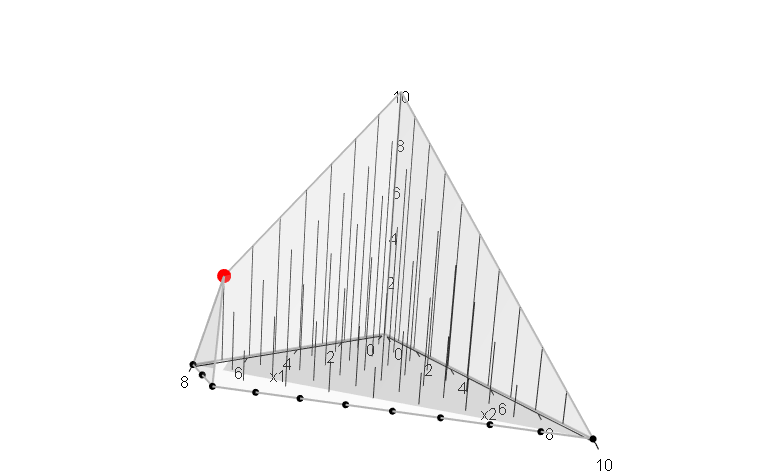MILP model with variable 1 integer:

loadView(v = view, zoom = 0.75)
plotPolytope(A, b, type = c("i","c","c"), plotOptimum = TRUE, obj = obj, plotFaces = FALSE)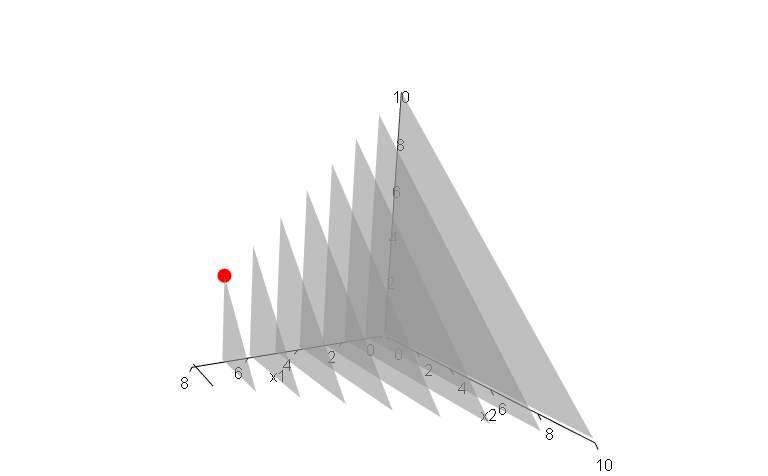MILP model with variable 2 integer:

loadView(v = view, zoom = 0.75)
plotPolytope(A, b, type = c("c","i","c"), plotOptimum = TRUE, obj = obj, plotFaces = FALSE)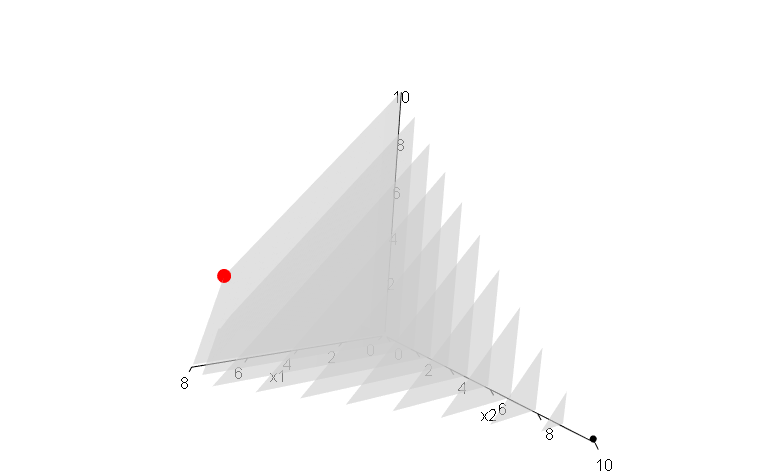MILP model with variable 3 integer:

loadView(v = view, zoom = 0.75)
plotPolytope(A, b, type = c("c","c","i"), plotOptimum = TRUE, obj = obj, plotFaces = FALSE)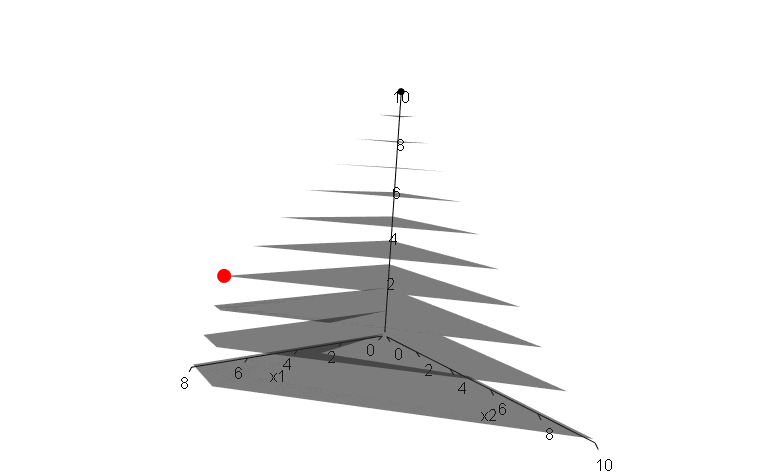## Example 3

We define the model:

A <- matrix( c(
-1, 1, 0,
1, 4, 0,
2, 1, 0,
3, -4, 0,
0, 0, 4
), nc = 3, byrow = TRUE)
b <- c(5, 45, 27, 24, 10)
obj <- c(5, 45, 15)

We load the preferred view angle for the RGL window:

view <- matrix( c(0.976349174976349, -0.202332556247711, 0.0761845782399178, 0, 0.0903248339891434,
0.701892614364624, 0.706531345844269, 0, -0.196427255868912, -0.682940244674683,
0.703568696975708, 0, 0, 0, 0, 1), nc = 4)

The LP polytope:

loadView(v = view, close = F, zoom = 0.75)
plotPolytope(A, b, plotOptimum = TRUE, obj = obj, labels = "coord")

Note in the html file you can zoom/turn/twist the figure with your mouse (rglwidget).

The ILP model with LP and ILP faces:

loadView(v = view)
mfrow3d(nr = 1, nc = 2, sharedMouse = TRUE)
plotPolytope(A, b, faces = c("c","c","c"), type = c("i","i","i"), plotOptimum = TRUE, obj = obj,
argsTitle3d = list(main = "With LP faces"), argsPlot3d = list(box = F, axes = T) )
plotPolytope(A, b, faces = c("i","i","i"), type = c("i","i","i"), plotFeasible = FALSE, obj = obj,
argsTitle3d = list(main = "ILP faces") )

Let us have a look at some MILP models (we use static images). MILP model with variable 1 and 3 integer:

loadView(v = view, close = T, zoom = 0.75)
plotPolytope(A, b, faces = c("c","c","c"), type = c("i","c","i"), plotOptimum = TRUE, obj = obj)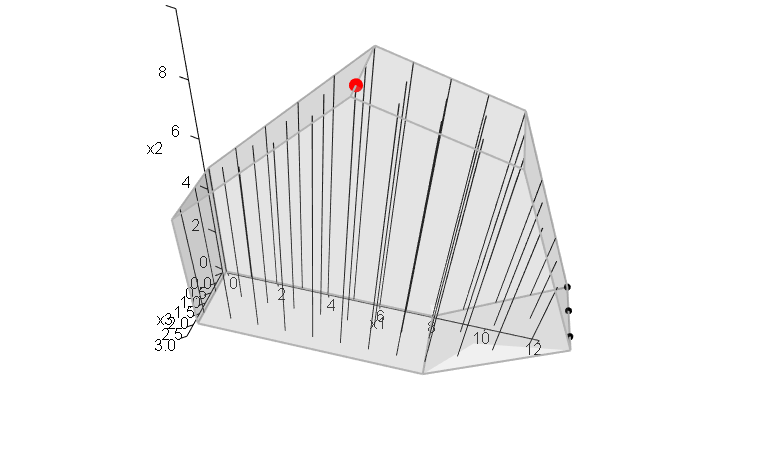MILP model with variable 2 and 3 integer:

loadView(v = view, zoom = 0.75)
plotPolytope(A, b, faces = c("c","c","c"), type = c("c","i","i"), plotOptimum = TRUE, obj = obj)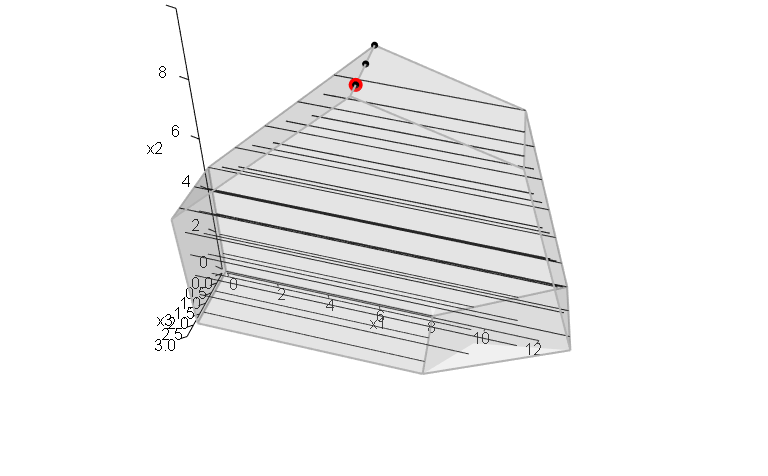MILP model with variable 1 and 2 integer:

loadView(v = view, zoom = 0.75)
plotPolytope(A, b, faces = c("c","c","c"), type = c("i","i","c"), plotOptimum = TRUE, obj = obj)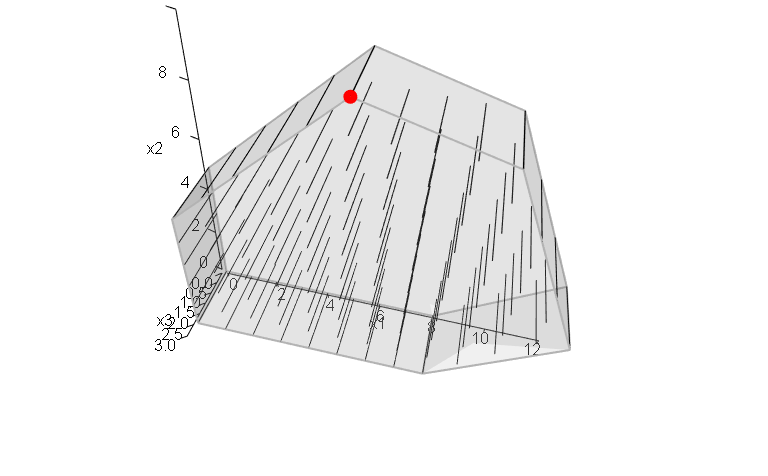MILP model with variable 1 integer:

loadView(v = view, zoom = 0.75)
plotPolytope(A, b, type = c("i","c","c"), plotOptimum = TRUE, obj = obj, plotFaces = FALSE)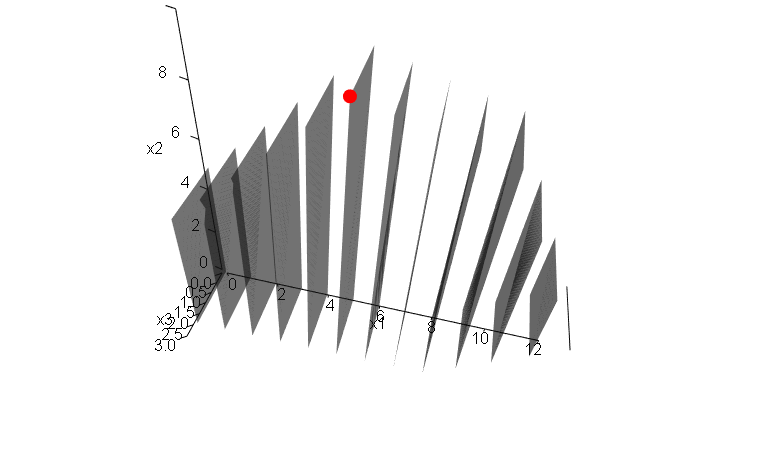MILP model with variable 2 integer:

loadView(v = view, zoom = 0.75)
plotPolytope(A, b, type = c("c","i","c"), plotOptimum = TRUE, obj = obj, plotFaces = FALSE)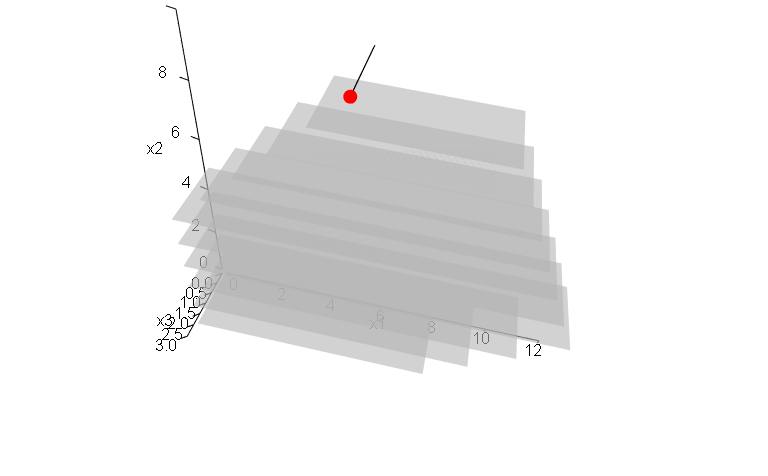MILP model with variable 3 integer:

loadView(v = view, zoom = 0.75)
plotPolytope(A, b, type = c("c","c","i"), plotOptimum = TRUE, obj = obj, plotFaces = FALSE)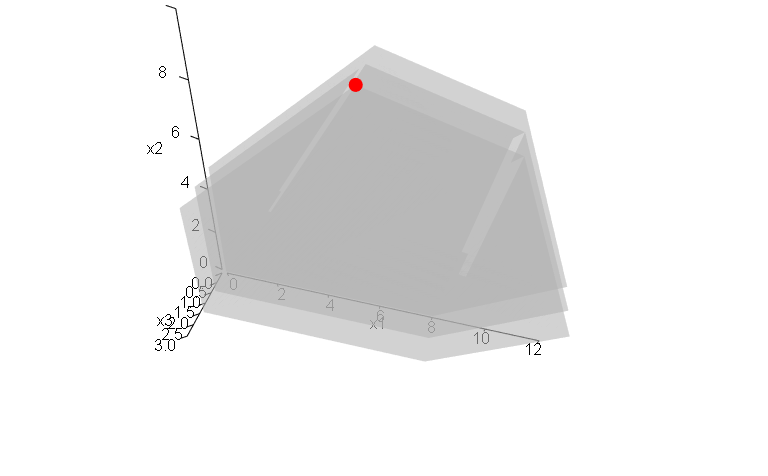## Example 4

We define the model:

Ab <- matrix( c(
1, 1, 2, 5,
2, -1, 0, 3,
-1, 2, 1, 3,
0, -3, 5, 2
#   0, 1, 0, 4,
#   1, 0, 0, 4
), nc = 4, byrow = TRUE)
A <- Ab[,1:3]
b <- Ab[,4]
obj = c(1,1,3)

We load the preferred view angle for the RGL window:

view <- matrix( c(-0.452365815639496, -0.446501553058624, 0.77201122045517, 0, 0.886364221572876,
-0.320795893669128, 0.333835482597351, 0, 0.0986008867621422, 0.835299551486969,
0.540881276130676, 0, 0, 0, 0, 1), nc = 4)

The LP polytope:

loadView(v = view, close = F, zoom = 0.75)
plotPolytope(A, b, plotOptimum = TRUE, obj = obj, labels = "coord")

Note in the html file you can zoom/turn/twist the figure with your mouse (rglwidget).

The ILP model with LP and ILP faces:

loadView(v = view)
mfrow3d(nr = 1, nc = 2, sharedMouse = TRUE)
plotPolytope(A, b, faces = c("c","c","c"), type = c("i","i","i"), plotOptimum = TRUE, obj = obj,
argsTitle3d = list(main = "With LP faces"), argsPlot3d = list(box = F, axes = T) )
plotPolytope(A, b, faces = c("i","i","i"), type = c("i","i","i"), plotFeasible = FALSE, obj = obj,
argsTitle3d = list(main = "ILP faces") )

Let us have a look at some MILP models (we use static images). MILP model with variable 1 and 3 integer:

loadView(v = view, close = T, zoom = 0.75)
plotPolytope(A, b, type = c("i","c","i"), plotOptimum = TRUE, obj = obj)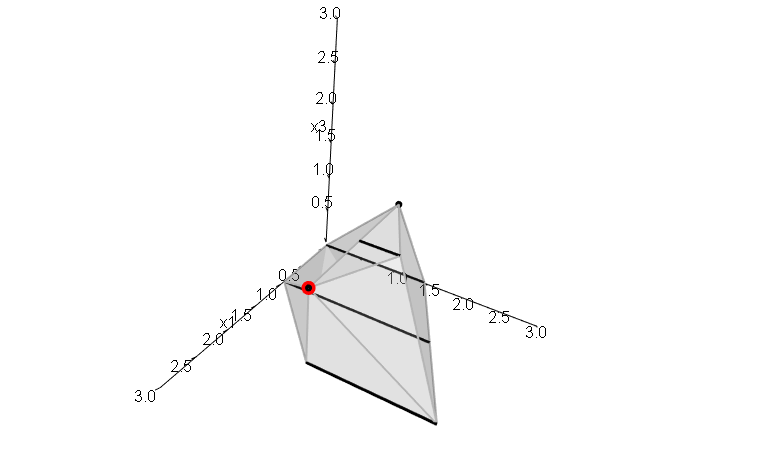MILP model with variable 2 and 3 integer:

loadView(v = view, zoom = 0.75)
plotPolytope(A, b, type = c("c","i","i"), plotOptimum = TRUE, obj = obj)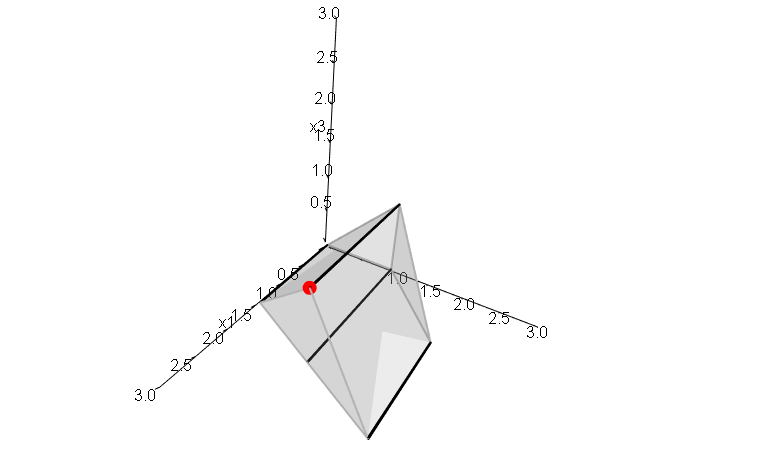MILP model with variable 1 and 2 integer:

loadView(v = view, zoom = 0.75)
plotPolytope(A, b, type = c("i","i","c"), plotOptimum = TRUE, obj = obj)